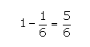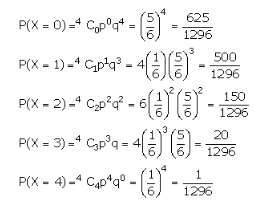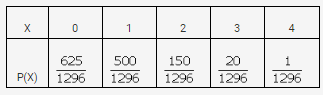# A pair of dice is thrown 4 times. If getting a doublet is considered

A pair of dice is thrown 4 times. If getting a doublet is considered a success, find the probability distribution of the number of successes.

Total number of outcomes = 36
The possible doublets are (1, 1), (2, 2), (3, 3), (4, 4), (5, 5), and (6, 6).
Let p be the probability of success, therefore,
p =So, q = 1 - p =Since the dice is thrown 4 times, n=4
Let X denote the number of times of getting doublets in the experiment of throwing two dice simultaneously four times.
Therefore X can take the values 0, 1, 2, 3, or 4.Thus, the probability distribution is: